# Why do plano-convex lenses reduce spherical aberration?

Mar. 23, 2022

I don't understand why the flat side would change the amount of spherical aberration if the focal length still has to be the same?

## Spherical Aberration in a Lens

If a point object is placed on the axis of the large lens, images Ip and Im will be formed by the paraxial and marginal rays respectively. The paraxial rays of light from the image are at a longer distance from the lens than the marginal rays. The image is not sharp at any point on the axis. If the screen is placed perpendicular to the axis at AB, the image appears to be a circular patch of diameter AB. The patch of diameter AB is called the circle of least confusion and corresponds to the position of the best image. The distance Imap measures the longitudinal spherical aberration and the radius of the circle of least confusion measures the lateral spherical aberration. When the aperture of the lens is relatively large compared to the focal length of the lens, the cones of the rays of light refracted through different zones of the lens surface are not brought to focus at the same point on the axis. The marginal rays come to focus at a nearer point Im and the paraxial rays come to focus at a farther point Ip. Thus for point object O on the axis, the image extends over the length ImIp . This effect is called spherical aberration and arises due to the fact that different annular zones have different focal lengths.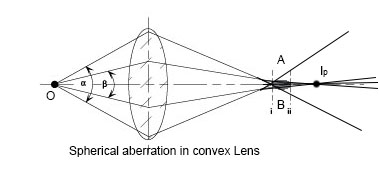The spherical aberration produced by a concave lens is shown in the figure. It is positive for the convex lens and negative for the concave lens, both paraxial and marginal rays cannot focus at a single point. The rays incident on the lens near the principal axis are called paraxial rays and the rays falling near the edge are called marginal rays.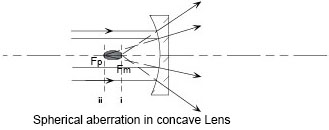## Removal of Spherical Aberration in lenses

For a single lens, spherical aberration cannot be entirely eliminated. However, it can be reduced by the following methods:

1. By using stop
Spherical aberration can be reduced either by cutting off the paraxial rays or by cutting off the marginal rays.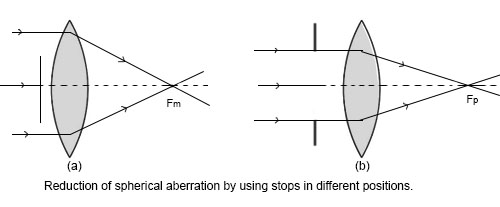2. By using plano-convex lenses
If parallel rays of light incident on the plane surface of the plano-convex lens, the spherical aberration will be maximum because incident rays entire deviation at the convex surface. Similarly if parallel rays of light incident on the convex surface, spherical aberration will be minimum.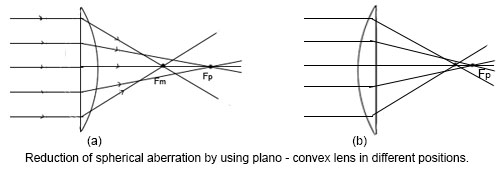A telescope objective receives parallel rays of light from a distant object. To reduce spherical aberration, the convex surface of the plano-convex lens is always towards the distant object. In a microscope objective, the rays fall on it from a very near point object and hence the incident rays are bound to be much oblique than the emergent rays. If the convex surface is towards the object, spherical aberration will be maximum and will be minimum if the plane surface faces the object.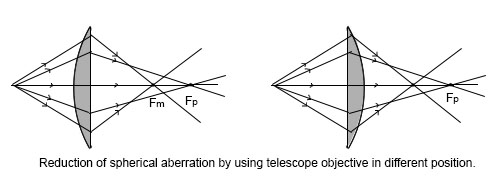3. By using two lenses separated by a distance
When two convergent lenses, separated by a distance are used, the refraction takes place at four surfaces. The spherical aberration will be least when there is an equal deviation at all a surface. It is achieved if,

f2–F1=df2–F1=d

4. where f2 and f1 are the focal length of the two lenses and d is distance between them. This arrangement is used in eye-pieces.

5. By combining suitable concave and convex lenses
It is known that convex lens has positive spherical aberration and concave lens has negative spherical aberration. So by selecting suitable pair of concave and convex lens, spherical aberration can be minimised.